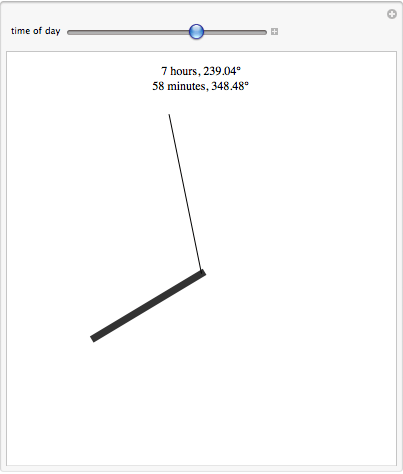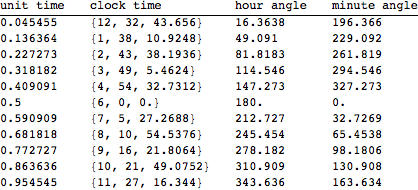Opposing clock hands

I was recently sitting in a poorly produced play called That Hopey Changey Thing when, looking wistfully at my watch, I noticed that the hour hand and the minute hand were perfectly lined up, pointing in opposite directions. I stayed awake for the rest of the show in part thanks to the thought puzzle of how many times a day this happens, and how best to determine exactly when.

First, I found it useful to think of time in terms of a unit clock cycle — the hands on our theoretical clock run from 0 to 1, rather than to 12. The following Manipulate[] command draws a clock, allowing the user to set the unit time, and translating it into both conventional time and the angle of the minute and hour hands.

` In[]:= Manipulate[ Graphics[{{Opacity, Disk[{0, 0}, .9]}, {Opacity[.8], Thickness[.02], Line[{{0, 0}, .65 {Sin[2 Pi m], Cos[2 Pi m]}}]}, Line[{{0, 0}, .85 {Sin[12*2 Pi m], Cos[12*2 Pi m]}}]}, PlotLabel -> ToString[Floor[Mod[11 + m*12, 12] + 1]] <> " hours, " <> ToString[360.*m] <> "\[Degree]\n" <> ToString[Floor[Mod[m, 1/12]*720]] <> " minutes, " <> ToString[4320 Mod[m, 1/12]] <> "\[Degree]"], {{m, 0, "time of day"}, 0, 1}] `

The code when running looks likeThis was useful when debugging the formulae for the conversions; any error was immediately apparent.

Breaking out the conversions explicitly, we have

` In[]:= unitTimeToListTime[unitTime_Real] := Module[{hour, minute, seconds}, hour = Floor[Mod[11 + unitTime*12, 12] + 1]; minute = Floor[Mod[unitTime, 1/12]*720]; seconds = Mod[unitTime, 1/720]*60*720; {hour, minute, seconds} ] `
`In[]:= unitTimeToMinuteAngle[unitTime_Real] := 4320 Mod[unitTime, 1/12]`

and

`In[]:= unitTimeToHourAngle[unitTime_Real] := unitTime*360 // N`

These in hand, I naively thought the problem was as good as solved. I defined my problem in functional form,

`In[]:= unstraightness[unitTime_] := Abs[Abs[unitTimeToMinuteAngle[unitTime] - unitTimeToHourAngle[unitTime]] - 180]`

checked it with

`Plot[unstraightness[unitTime], {unitTime, 0, .999999}]`and we see as expected a periodic function. There are 11 solutions when “unstraightness” == 0 per day, which makes perfectly good sense when you think about it.

I thought I could determine these with `NSolve[unstraightness[unitTime] == 0, unitTime]`

but sadly, this yields nothing but error messages.
`NSolve::nsmet: This system cannot be solved with the methods available to NSolve. >>`

What to do? I decided on brute force. Slice the day into 1,000,000 pieces and identify the 11 times which produce the most opposed clock hands.

`In[]:= handangle = Table[{unitTime, Abs[unitTimeToMinuteAngle[unitTime] - unitTimeToHourAngle[unitTime]]}, {unitTime, 0, .999999, .000001}];`

`In[]:= closeFits = Select[handangle, Abs[#[] - 180] < .002 &];`

```In[]:= TableForm[ Map[{#, ToString[unitTimeToListTime[#]], unitTimeToHourAngle[#], unitTimeToMinuteAngle[#]} &, Transpose[closeFits][]], TableHeadings -> {None, {"unit time", "clock time", "hour angle", "minute angle"}}]```Ah-hah!

As looked to be the case from the graph, these solutions are clearly spaced exactly 1/11 of the clock apart from each other. This means that we can get a more perfect solution more quickly with

`In[]:= Table[ToString[unitTimeToListTime[i // N][]] <> ":" <> ToString[unitTimeToListTime[i // N][]] <> ":" <> ToString[unitTimeToListTime[i // N][]], {i, 1/22, .9999, 1/11}] // TableForm`

which returns

12:32:43.6364
1:38:10.9091
2:43:38.1818
3:49:5.45455
4:54:32.7273
6:00:00.
7:5:27.2727
8:10:54.5455
9:16:21.8182
10:21:49.0909
11:27:16.3636

Clearly, my bored glance at my watch had been at 8:10:54 pm.

One of the nice things about this solution method is that it easily generalizes to solve other “clock face” problems. For example, in a second we can determine that the hands of a clock are perfectly lined up, facing the same direction, at the following times:
12:00:00.
1:5:27.2727
2:10:54.5455
3:16:21.8182
4:21:49.0909
5:27:16.3636
6:32:43.6364
7:38:10.9091
8:43:38.1818
9:49:5.45455
10:54:32.7273

One thought on “Opposing clock hands”

1. Pingback: clock hands, part ii | monkeywrench